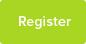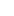# Tutor Hunt Questions

A length of cable and the drum it is wrapped around have a combined mass of 50kg. The mass of the drum is a quarter the mass of the cable. Removing 35m of cable leaves the drum as one third the remaining mass of the cable.

2 years ago

Mass of cable = x Mass of drum = 0.25x x+0.25x=50 1.25x=50 x=50/1.25 =40 Removing 35m of cable = x-35=40-35=5 x-35 = 1/3*x
10/11/2016 07:41:59 | comment by Viswanath

Please enter your response to the question below. The student will get a notification as soon your response has been approved by our moderation team.

Let m = mass of drum, let M be original mass of cable:

can form a simultaneous pair..

m + M = 50 [kg]

m = M/4

solving gives 5M/4 = 50 so mass of cable is 40kg
m mass of drum is 10kg

Take away 35m of cable now have..
m = M`/3 where M` is new reduced mass of cable remaining, M` = 30kg

Cable say length L has mass 40kg, (L-35)has mass 30kg, can form proportion: (L-35}/L = 30/40 = 3/4
so solving for L gives, 1-(35/L) = 3/4 or 35/L = 1/4 hence L = 140,

i.e. original length of cable is 140m.

Answered by Tom | 2 years ago
Let mass of drum be "D"

Original mass of cable therefore = 4D

Mass of shortened cable = 3D

So a quarter of the mass (and therefore a quarter of the length) of the cable has been removed,

so the original length of the cable was 140m

Answered by Laura | 2 years ago
M_d is the mass of the drum. M_c is the original mass of the cable. M_cnew is the new mass of the cable.

M_d + M_c = 50 <1>
M_d=0.25*M_c <2>

Put <2> into <1>
M_d + M_c = 50
0.25*M_c + M_c = 50
so 1.25*M_c = 50
so M_c = 50/1.25 = 40

Put this into <1>
M_d + M_c = 50
M_d + 40 = 50
so M_d = 10

So know we know the drum originally weighed 10kg, the cable 40kg.

M_d = M_cnew / 3
so M_cnew = 3 * M_d = 3 * 10 = 30kg

So original cable weighed 40kg. Now weighs 30kg having had 35m chopped off. So 35m of cable weighed (40-30)=10kg. So 40kg of cable (original length weighed this much) is 4 times as long, i.e. 35m * 4 = 140m.

(Done in a hurry! Will check later!)
Hello,

I tried to write a much more detailed explanation but there wasn`t enough room in the box. If you have any more questions then ask.

The answer that I got was 140m.

I got this by

1) Calculating the mass of the drum and cable originally by combining that fact that we know the combined mass of the drum and cable, and the fact that the drum is a quarter the mass of the cable originally. Mass of drum = 10kg and mass of cable is 40 kg.

2) Understand that you need to work out the density per unit length of the cable p by using the equation M=pL, where M is mass and L is length. You can do this by knowing that when the length of the cable is reduced the mass of the drum is now a third the mass of the cable and the fact that we know that the mass of the cable originally is the sum of the mass of the cable when it`s 35 m and the remaining length of the cable.

3) Do a load of substitutions to get the mass of the cable when it`s 35 m long, then you can substitute this into the equation in 2) to get the density per unit length.

4) From this you can also calculate the mass of the remaining bit of cable, which should be 30 kg. You should have calculated p = 10/35 kg/m. Then from M=pL, Lr=M/p=30/(10/35)=105 m.

5) Obviously L = Lr + L35. So L = 105+35=140m